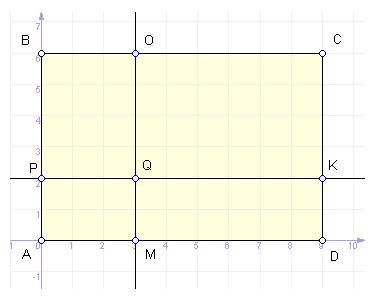bolt
Try our new interface for solving problems
Problems

# Four Square

Time limit 1 second
Memory limit 64 MiBDirect x = a and y = b divide the quadrilateral with vertices A(0, 0), B(0; y1), C(x1; y1), D(x1; 0) into four parts. Determine the square formed by the figures.

## Input data

In a single line through the space defined four integers: a, b, x1 and y1. All input data for the module does not exceed 100.

## Output data

Print the first area of a quadrilateral APQM, then through the gap - PBOQ, then through the gap - QOCK, and, finally, through the gap - MQKD. The results lead to three decimal.

## Examples

Input example #1
3 2 9 6


Output example #1
6.000 12.000 24.000 12.000

Input example #2
-13 2 -9 6

Output example #2
26.000 52.000 16.000 8.000